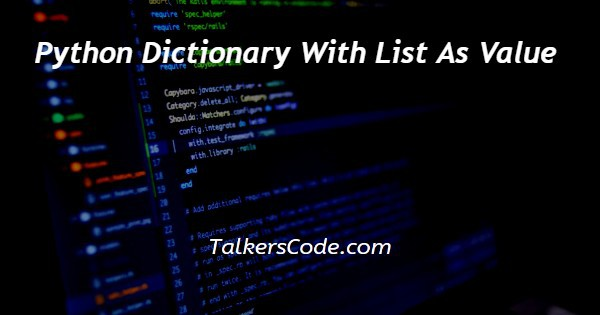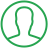# Python Dictionary With List As Value

Last Updated : Jul 1, 2023In this tutorial we will show you the solution of python dictionary with list as value, in the Python dictionary, it is an unordered and immutable data type and can be used as a key element.

In Python, the dictionary of lists means we have to assign values as a list. So, let’s get started.

## Step By Step Guide On Python Dictionary With List As Value :-

Python supports two ways of creating a dictionary :

• Using {} or dict()
• Using the built-in zip() function

Creating an empty dictionary and adding key:value pairs to it

```dict1 = {'A':[11, '2', 53], 'B':[4, 50]}
print(dict1)
print(dict1['A'])
print(dict1['A'])
dict2 = dict(X = [5, 7, 9], Y = [41, 15])
print(dict2)
keysList = ['A', 'B', 'C']
valuesList = [[7, 1, 5], [17, 9, 18], [11, 1]]
dict1 = dict(zip(keysList, valuesList))
print(dict1)
dict1 = {}
dict1['A'] = [1, 2, 3]
dict1['B'] = [10, 12]
print(dict1)```
1. In the first line, there is a variable named dict1 which holds the values as lists.
2. You can pass the lists directly within a {} pair and it will create a dictionary by default.
3. There are two lists that are passed as the value for creating a dictionary. The list’s names are ‘A’ and ‘B’.
4. In the next line, there is a print statement that will print the value of the variable dict1.
5. Now in the next two lines, there are two print statements that describe how to access any element from a dictionary.
6. The dict1[‘A’] will print the whole list of values that the list ‘A’ contains. And the dict1[‘A’] will print the first element of the list ‘A’. Here it is 11.
7. In the next approach, we will use the dict() function to create a dictionary. You just have to pass the list name and the list values as the arguments in this function.
8. In the next line, there is a variable named dict2 which will become a dictionary after the returned value from the dict() function.
9. In this case, this dictionary will have two lists as the values of the dictionary. In the next line there is a print statement that will print the value of the variable dict2.
10. In the next approach, you can use a built-in function called the zip() function to create a dictionary that contains lists as values.
11. In the next line there is a variable named keysList, which is a list that contains three character values: ‘A’, ‘B’, and ‘C’. These characters will be treated as the names of the list.
12. In the next line, there is another variable named valuesList that is a list that contains three list values.
13. In the next line, there is a variable named dict1 that will become a dictionary as a result of the call of the dict() function that has the zip() function passed as the argument.
14. The zip() function accepts two parameters. The first one is the name of the lists. Here it is the values of the variable named keysList.
15. And the second one is the values of the lists that you want to include in the dictionary. In the next line, there is a print statement that will print the value of the variable dict1.
16. In the next line, there is another variable named dict1, which is an empty dictionary. You can create an empty dictionary by using the {}.
17. Now you can insert any number of lists as the values of the dictionary. In this case, there are two lists: list ‘A’ and list ‘B’, which will be inserted into the dictionary.
18. In the last line, there is a print statement that will print the values of the variable dict1.

## Conclusion :-

So finally, in conclusion, we can say that with the help of this tutorial, you can now create a dictionary that has lists as values in it and access the elements of a dictionary in a Python program.

You can use any of the methods mentioned above that you find easy to understand.

I hope this tutorial on python dictionary with list as value helps you and the steps and method mentioned above are easy to follow and implement.

##About Pragati

Experienced coding content writer who enjoys breaking down complex concepts in programming languages like Java, Python, C, and C++. Over three years of experience producing interesting and relevant content for a variety of entities. Committed to providing concise and easy-to-understand articles that assist readers in easily understanding technology and industry trends in the world of coding and software development.